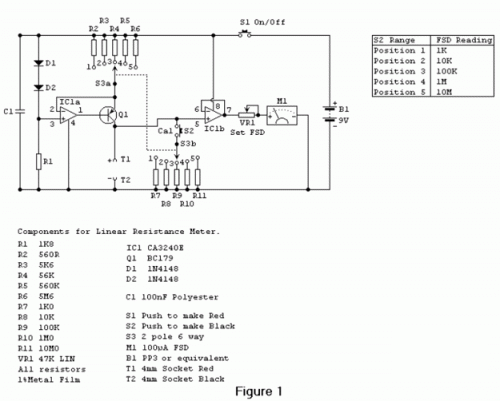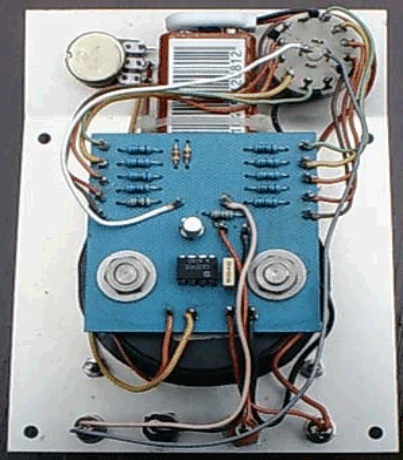# Electronic projects

you are here: home page :: projects :: Tools & measurement :: measuring devices :: Linear Resistance Meter

## Linear Resistance Meter

Most analogue multimeters are capable of measuring resistance over quite a wide range of values, but are rather inconvenient in use due to the reverse reading scale which is also non-linear. This can also give poor accuracy due to cramping of the scale that occurs at the high value end of each range. This resistance meter has 5 ranges and it has a forward reading linear scale on each range.The full-scale values of the 5 ranges are 1K, 10K, 100K, 1M &10M respectively and the unit is therefore capable of reasonably accurate measurements from a few tens of ohms to ten Megohms.

Circuit diagramThe Circuit
Most linear scale resistance meters including the present design, work on the principle that if a resistance is fed from a constant current source the voltage developed across that resistance is proportional to its value. For example, if a 1K resistor is fed from a 1 mA current source from Ohm’s Law it can be calculated that 1 volt will be developed across the resistor (1000 Ohms divided by 0.001 amps = 1 volt). Using the same current and resistance values of 100 ohms and 10K gives voltages of 0.1volts (100 ohms / 0.001amps = 0.1volts) and 10 volts (10000 ohms / 0.001amps = 10 volts).
Thus the voltage developed across the resistor is indeed proportional to its value, and a voltmeter used to measure this voltage can in fact be calibrated in resistance, and will have the desired forward reading linear scale. One slight complication is that the voltmeter must not take a significant current or this will alter the current fed to the test resistor and impair linearity. It is therefore necessary to use a high impedance voltmeter circuit.
The full circuit diagram of the Linear Resistance Meter is given in Figure 1. The constant current generator is based on IC1a and Q1. R1, D1 and D2 form a simple form a simple voltage regulator circuit, which feeds a potential of just over 1.2 volts to the non-inverting input of IC1a. There is 100% negative feedback from the emitter of Q1 to the inverting input of IC1a so that Q1’s emitter is stabilised at the same potential as IC1a’s non-inverting input. In other words it is stabilised a little over 1.2 volts below the positive supply rail potential. S3a gives 5 switched emitter resistances for Q1, and therefore 5 switched emitter currents. S3b provides 5 reference resistors across T1 & T2 via S2 to set full-scale deflection on each range using VR1.
As the emitter and collector currents of a high gain transistor such as a BC179 device used in the Q1 are virtually identical, this also gives 5 switched collector currents. By having 5 output currents, and the current reduced by a factor of 10 each time S3a is moved one step in a clockwise direction, the 5 required measuring ranges are obtained. R2 to R6 must be close tolerance types to ensure good accuracy on all ranges. The high impedance voltmeter section uses IC1b with 100% negative feedback from the output to the inverting input so that there is unity voltage gain from the non-inverting input to the output. The output of IC1b drives a simple voltmeter circuit using VR1 and M1, and the former is adjusted to give the correct full-scale resistance values.
The CA3240E device used for IC1 is a dual op-amp having a MOS input stage and a class A output stage. These enable the device to operate with the inputs and outputs right down to the negative supply rail voltage. This is a very helpful feature in many circuits, including the present one as it enables a single supply rail to be used where a dual balanced supply would otherwise be needed. In many applications the negative supply is needed simply in order to permit the output of the op-amp to reach the 0volt rail. In applications of this type the CA3240E device normally enables the negative supply to be dispensed with.
As the CA3240E has a MOS input stage for each section the input impedance is very high (about 1.5 million Megohms!) and obviously no significant input current flows into the device. This, together with the high quality of the constant current source, and the practically non-existent distortion through IC1b due to the high feedback level gives this circuit excellent linearity.
With no resistor connected across T1 & T2 M1 will be taken beyond full-scale deflection and overloaded by about 100 or 200%. This is unlikely to damage the meter, but to be on the safe side a push-to-test on/off switch (S1) is used. Thus the power is only applied to the circuit when a test resistor is connected to the unit, and prolonged meter overloads are thus avoided.
A small (PP3 size) 9 volt battery is a suitable power source for this project which has a current consumption of around 5mA and does not require a stabilised supply.

Photos showing inside and outside of the completed Linear Resistance Meter.author: Dave Elliott
e-mail: portagepal@tiscali.co.uk
web site: http://www.electronics-lab.com
project categories:Audio (49)Power & high voltage (43)Radio (23)Light & LED (31)Tools & measurement (40)Telephone (18)Automotive (10)Microcontrollers (12)Sensors & control (47)Timers & oscillators (40)Miscellaneous (26)

StumbleUpon button

project subcategories:
All subcategories
testing equipment
measuring devices
other tools

similar projects:
Capacitance Meter
Digital Remote Thermometer
Digital Voltmeter
Electromagnetic Field Probe with Meter Output
Field-strength meter
Field-strength meter
Led display digital Voltmeter
MILLIGAUSS METER.
Picoammeter circuit with 4 ranges

Doorbell for the Deaf
High Quality Intercom
Combinational Conjuring Trick
POT-PLANT POWER
Ultrasonic Dog Whistle
Quiz Circuit
LED Torch
Insect Repellant
Speaker Microphone Circuit
Magic Wand Conjuring Trick.

most popular projects:
Led display digital Voltmeter
500W low cost 12V to 220V inverter
Low pass filter - Subwoofer
Metal Detector
High And Low Voltage Cut Off With Time Delay
2N3055 Power Amplifier
Basic UPS Power Supply
200W audio amplifier
LED Torch
100W RMS Amplifier

DIY Electronics ProjectsPromote Your Page Too